Date: Tue, 29 Apr 1997 14:44:55 +0200
Sender: Peter

Subject: irrational numbers

Hello!

We have a little mathematical problem... we need some help proving e is an irrational number!

We don't feel very confident in our formulas, so if You have the time to give us a little explanation we would be very grateful!!!

Thank You very much!!

/"Students in trouble" (Peter and Jenny)

We asume that e is an irrational number, that e=(a/b) where a and b are positive "whole" numbers. We asume that e is an irrational number, that e=(a/b) where a and b are positive "whole" numbers. By using the Taylor-method for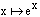around x = 0. For every positive "whole" number n there is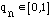so that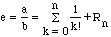,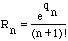We want to show that b*n!*Rn is a "whole" number for all n 1 and that b*n!*Rn 0 when

n approaches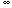.

This will be a contradiction. We wan't to know why!!

Hi Peter and Jenny

What you are doing here is correct but you don't need a special form for the remainder. It is more direct to write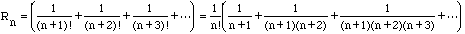since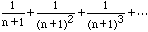is a geometric series. Thus when n=b,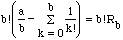is an integer but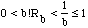which is impossible.

Cheers

Doug and Penny

Go to Math Central

To return to the previous page use your browser's back button.